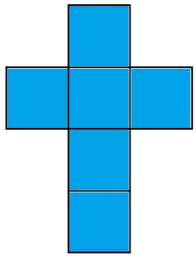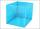# Cube V2S

The volume of the cube is 27 dm cubic.
Calculate the surface of the cube.

S =  54 dm2

### Step-by-step explanation:Did you find an error or inaccuracy? Feel free to write us. Thank you!Tips to related online calculators
Tip: Our volume units converter will help you with the conversion of volume units.

## Related math problems and questions:

• Cube surface and volumeFind the surface of the cube with a volume of 27 dm3.
• The cubeThe cube has a surface area of 486 m ^ 2. Calculate its volume.
• The cubeThe cube has a surface area of 216 dm2. Calculate: a) the content of one wall, b) edge length, c) cube volume.
• Third dimensionCalculate the third dimension of the cuboid: a) V = 224 m3, a = 7 m, b = 4 m b) V = 216 dm3, a = 9 dm, c = 4 dm
• The cubeThe cube has a surface of 600 cm2. What is its volume?
• Cube basicsHow long is the edge length of a cube with volume 15 m3?
• The volume 2The volume of a cube is 27 cubic meters. Find the height of the cube.
• Cube 5The surface of the cube is 15.36 dm2. How will change the surface area of this cube if the length of the edges is reduced by 2 cm?
• Area of a cubeCalculate the surface area of a cube if its volume is equal to 729 cubic meters.
• Cube surfce2volumeCalculate the volume of the cube if its surface is 150 cm2.
• TerezaThe cube has an area of base 256 mm2. Calculate the edge length, volume, and area of its surface.
• Cube edgeDetermine the edges of the cube when the surface is equal to 37.5 cm square.
• Cube 5The content area of one cube wall is 32 square centimeters. Determine the length of its edges, its surface and volume.
• Edges or sidesCalculate the cube volume, if the sum of the lengths of all sides is 276 cm.
• Area to volumeIf the surface area of a cube is 486, find its volume.
• Cuboid to cubeA cuboid with dimensions of 9 cm, 6 cm, and 4 cm has the same volume as a cube. Calculate the surface of this cube.
• Volume and areaWhat is the volume of a cube which has an area of 361 cm2?